Share

# The Three Rods Shown in Figure (28−E7) Have Identical Geometrical Dimensions. Heat Flows from the Hot End at a Rate of 40 W in the Arrangement (A). - Physics

ConceptThermal Expansion of Solids

#### Question

The three rods shown in figure  have identical geometrical dimensions. Heat flows from the hot end at a rate of 40 W in the arrangement (a). Find the rates of heat flow when the rods are joined as in arrangement (b) and in (c). Thermal condcutivities of aluminium and copper are 200 W m−1°C−1 and 400 W m−1°C−1 respectively.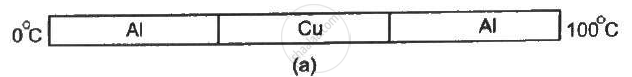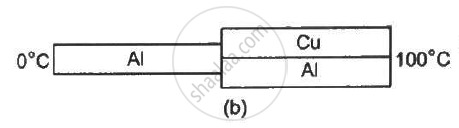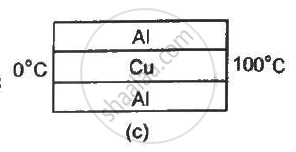#### Solution

For arrangement (a),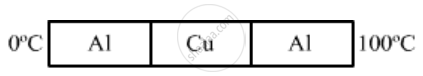Temperature of the hot end ,T1 = 100°C

Temperature of the cold end ,T2 = 0°C

R_s = R_1 + R_2 + R_3

(l)/(K_{AI}A)  + l/(K_{cu}A) + {l}/(K_{Al}A)

(l)/(A) ( 1/200 +1/400 + 1/200)

1/A (5/400)

1/Axx 1/80

(dQ)/d = q = Rate  of  flow  of  heat = (T_1 - T_2)/R_s

= (100 - 0)/((1/Axx1/(80))

Given :

q = 40W

40 = (100)/(1/Axx 1/80)

⇒ l/A = 200

⇒ l/A = 1/200

For arrangement (b),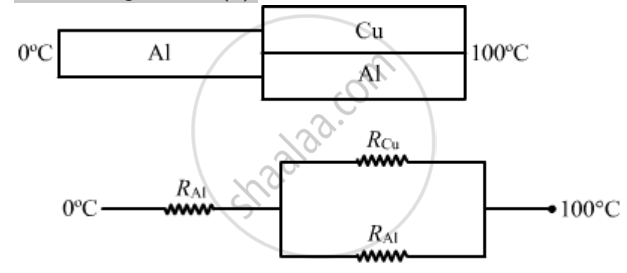"R_net" = R_[AI} + (R_cuxxR_{AI})/(R_cu = R_{AI)}

= l / (K_AI A) + (l/(K_cuA)xxl/K_(AI))/(l / (K_{cu}A9 )+ (l)/ (K_{AL}A)

= (l)/(A.K_Al) +( l )/ (A(K_AL + K_cu))

= l/A  (1/200 + 1/"200 + 400")

=l/A ( 1/200 + 1/600)

= 4/600 1/A

Rate of flow of heat is given by

q = (T_1 - T_2)/ R_"net"

= ((100 - 0))/ (4 l)   600 A

= (100xx600)/4 xx1/200

= 75 W

For arrangement (c),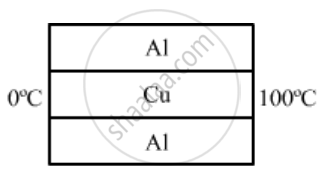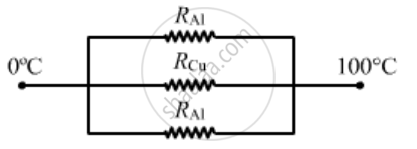1 / (R"net")  = (1)/(R_{AI)) + 1/R_(cu)+ 1/(R_AI)

= K_{K_{AI}}/ (l)+ K_(cuA)/l  + (K_{Al}A)/l

1/R_"net" = (K_Al + K_( cu) + K_(Al))A/l

l /R_"(net)" = (200 + 400 +200)

1/ R_"net" = 200/800

=(100)/(1/4)

rate of heat flow = (DeltaT)/R_"net"

= 100/1/4400 W

Is there an error in this question or solution?

#### APPEARS IN

Solution The Three Rods Shown in Figure (28−E7) Have Identical Geometrical Dimensions. Heat Flows from the Hot End at a Rate of 40 W in the Arrangement (A). Concept: Thermal Expansion of Solids.
S